# Stochastic matrix

(diff) ← Older revision | Latest revision (diff) | Newer revision → (diff)

A square (possibly infinite) matrix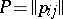with non-negative elements, for which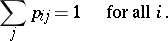The set of all stochastic matrices of orderis the convex hull of the set of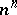stochastic matrices consisting of zeros and ones. Any stochastic matrixcan be considered as the matrix of transition probabilities of a discrete Markov chain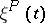.

The absolute values of the eigenvalues of stochastic matrices do not exceed 1; 1 is an eigenvalue of any stochastic matrix. If a stochastic matrixis indecomposable (the Markov chainhas one class of positive states), then 1 is a simple eigenvalue of(i.e. it has multiplicity 1); in general, the multiplicity of the eigenvalue 1 coincides with the number of classes of positive states of the Markov chain. If a stochastic matrix is indecomposable and if the class of positive states of the Markov chain has period, then the set of all eigenvalues of, as a set of points in the complex plane, is mapped onto itself by rotation through an angle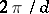. When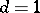, the stochastic matrixand the Markov chainare called aperiodic.

The left eigenvectors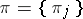ofof finite order, corresponding to the eigenvalue:(1)

and satisfying the conditions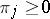,, define the stationary distributions of the Markov chain; in the case of an indecomposable matrix, the stationary distribution is unique.

Ifis an indecomposable aperiodic stochastic matrix of finite order, then the following limit exists: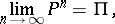(2)

where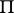is the matrix all rows of which coincide with the vector(see also Markov chain, ergodic; for infinite stochastic matrices, the system of equations (1) may have no non-zero non-negative solutions that satisfy the condition; in this caseis the zero matrix). The rate of convergence in (2) can be estimated by a geometric progression with any exponentthat has absolute value greater than the absolute values of all the eigenvalues ofother than 1.

Ifis a stochastic matrix of order, then any of its eigenvaluessatisfies the inequality (see ):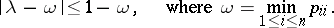The union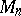of the sets of eigenvalues of all stochastic matrices of orderhas been described (see ).

A stochastic matrixthat satisfies the extra condition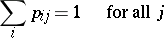is called a doubly-stochastic matrix. The set of doubly-stochastic matrices of orderis the convex hull of the set ofpermutation matrices of order(i.e. doubly-stochastic matrices consisting of zeros and ones). A finite Markov chainwith a doubly-stochastic matrixhas the uniform stationary distribution.

How to Cite This Entry:
Stochastic matrix. Encyclopedia of Mathematics. URL: http://encyclopediaofmath.org/index.php?title=Stochastic_matrix&oldid=15634
This article was adapted from an original article by A.M. Zubkov (originator), which appeared in Encyclopedia of Mathematics - ISBN 1402006098. See original article